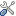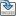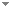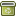# Enumerations of the Kolmogorov Function

Journal of Symbolic Logic 71 (2):501 - 528 (2006)

 Authors Piotr Grabowski Pedagogical University of Krakow Leen Torenvliet University of Amsterdam Harry Buhrman University of Amsterdam Abstract A recursive enumerator for a function h is an algorithm f which enumerates for an input x finitely many elements including h(x), f is a k(n)-enumerator if for every input x of length n, h(x) is among the first k(n) elements enumerated by f. If there is a k(n)-enumerator for h then h is called k(n)-enumerable. We also consider enumerators which are only A-recursive for some oracle A. We determine exactly how hard it is to enumerate the Kolmogorov function, which assigns to each string x its Kolmogorov complexity: • For every underlying universal machine U, there is a constant a such that C is k(n)-enumerable only if k(n) ≥ n/a for almost all n. • For any given constant k, the Kolmogorov function is k-enumerable relative to an oracle A if and only if A is at least as hard as the halting problem. • There exists an r.e., Turing-incomplete set A such for every non-decreasing and unbounded recursive function k, the Kolmogorov function is k(n)-enumerable relative to A. The last result is obtained by using a relativizable construction for a nonrecursive set A relative to which the prefix-free Kolmogorov complexity differs only by a constant from the unrelativized prefix-free Kolmogorov complexity. Although every 2-enumerator for C is Turing hard for K, we show that reductions must depend on the specific choice of the 2-enumerator and there is no bound on the quantity of their queries. We show our negative results even for strong 2-enumerators as an oracle where the querying machine for any x gets directly an explicit list of all hypotheses of the enumerator for this input. The limitations are very general and we show them for any recursively bounded function g: • For every Turing reduction M and every non-recursive set B, there is a strong 2-enumerator f for g such that M does not Turing reduce B to f. • For every non-recursive set B, there is a strong 2-enumerator f for g such that B is not wtt-reducible to f. Furthermore, we deal with the resource-bounded case and give characterizations for the class ${\rm S}_{2}^{{\rm P}}$ introduced by Canetti and independently Russell and Sundaram and the classes PSPACE, EXP. • ${\rm S}_{2}^{{\rm P}}$ is the class of all sets A for which there is a polynomially bounded function g such that there is a polynomial time tt-reduction which reduces A to every strong 2-enumerator for g. • PSPACE is the class of all sets A for which there is a polynomially bounded function g such that there is a polynomial time Turing reduction which reduces A to every strong 2-enumerator for g. Interestingly, g can be taken to be the Kolmogorov function for the conditional space bounded Kolmogorov complexity. • EXP is the class of all sets A for which there is a polynomially bounded function g and a machine M which witnesses A ∈ PSPACEf for all strong 2-enumerators f for g. Finally, we show that any strong O(log n)-enumerator for the conditional space bounded Kolmogorov function must be PSPACE-hard if P = NP Keywords No keywords specified (fix it) Categories Logic and Philosophy of Logic (categorize this paper) DOI 10.2178/jsl/1146620156 OptionsEdit this recordMark as duplicateExport citationFind it on ScholarRequest removal from indexRevision history

PhilArchive copy

Upload a copy of this paper     Check publisher's policy     Papers currently archived: 70,008

Setup an account with your affiliations in order to access resources via your University's proxy server
Configure custom proxy (use this if your affiliation does not provide a proxy)

## References found in this work BETA

Reducibility and Completeness for Sets of Integers.Richard M. Friedberg & Hartley Rogers - 1959 - Zeitschrift fur mathematische Logik und Grundlagen der Mathematik 5 (7-13):117-125.
Reducibility and Completeness for Sets of Integers.Richard M. Friedberg & Hartley Rogers - 1959 - Mathematical Logic Quarterly 5 (7‐13):117-125.
Effective Search Problems.Martin Kummer & Frank Stephan - 1994 - Mathematical Logic Quarterly 40 (2):224-236.

## Citations of this work BETA

Benign Cost Functions and Lowness Properties.Noam Greenberg & André Nies - 2011 - Journal of Symbolic Logic 76 (1):289 - 312.

## Similar books and articles

Kolmogorov Complexity for Possibly Infinite Computations.Verónica Becher & Santiago Figueira - 2005 - Journal of Logic, Language and Information 14 (2):133-148.
On the Complexity-Relativized Strong Reducibilites.Jari Talja - 1983 - Studia Logica 42 (2-3):259 - 267.
Using Biased Coins as Oracles.Toby Ord & Tien D. Kieu - 2009 - International Journal of Unconventional Computing 5:253-265.
Degrees of Monotone Complexity.William C. Calhoun - 2006 - Journal of Symbolic Logic 71 (4):1327 - 1341.
Every 2-Random Real is Kolmogorov Random.Joseph S. Miller - 2004 - Journal of Symbolic Logic 69 (3):907-913.
Non-Archimedean Probability.Vieri Benci, Leon Horsten & Sylvia Wenmackers - 2013 - Milan Journal of Mathematics 81 (1):121-151.